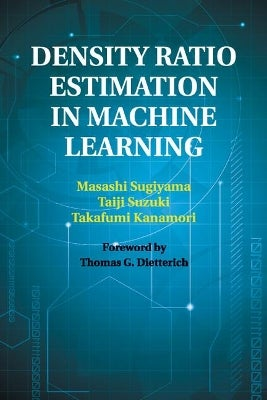•Free Click & Collect
•Free delivery on orders over \$50
•on orders of \$50 or more# Density Ratio Estimation in Machine Learning

By Masashi Sugiyama, Taiji Suzuki, Takafumi Kanamori

\$87.20

Or 4 payments of \$21.80 with

Delivered in 10 - 14 days
Available for Click and Collect

#### Toggle filter item Description

Machine learning is an interdisciplinary field of science and engineering that studies mathematical theories and practical applications of systems that learn. This book introduces theories, methods and applications of density ratio estimation, which is a newly emerging paradigm in the machine learning community. Various machine learning problems such as non-stationarity adaptation, outlier detection, dimensionality reduction, independent component analysis, clustering, classification and conditional density estimation can be systematically solved via the estimation of probability density ratios. The authors offer a comprehensive introduction of various density ratio estimators including methods via density estimation, moment matching, probabilistic classification, density fitting and density ratio fitting, as well as describing how these can be applied to machine learning. The book provides mathematical theories for density ratio estimation including parametric and non-parametric convergence analysis and numerical stability analysis to complete the first and definitive treatment of the entire framework of density ratio estimation in machine learning.

Product code: 9781108461733

ISBN 9781108461733 341 H230xW153xS18 Cambridge University Press

#### Toggle filter item Synopsis

Machine learning is an interdisciplinary field of science and engineering that studies mathematical theories and practical applications of systems that learn. The book introduces theories, methods and applications of density ratio estimation. This is the first and definitive treatment of the entire framework of density ratio estimation.